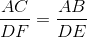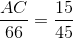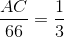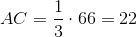# ISEE Upper Level Math : How to find if two acute / obtuse triangles are similar

## Example Questions

### Example Question #1 : Acute / Obtuse Triangles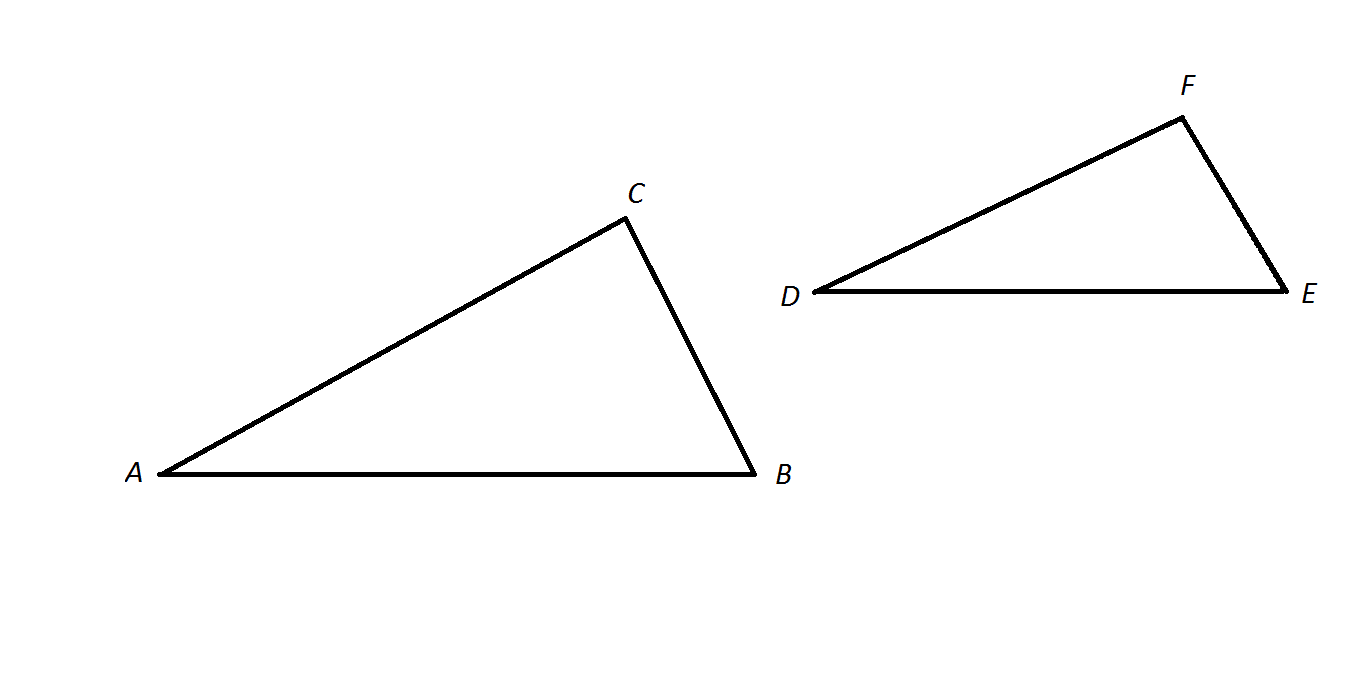NOTE: Figures NOT drawn to scale.

Refer to the above two triangles.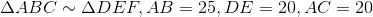What is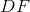?Insufficient information is given to answer the question.Explanation:

Corresponding sides of similar triangle are proportional, so if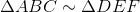, then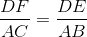Substitute the known sidelengths, then solve for: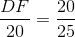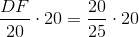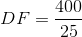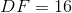### Example Question #81 : Isee Upper Level (Grades 9 12) Mathematics Achievement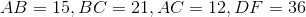What is the perimeter of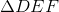?

It cannot be determined from the information given.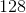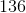Explanation:

By definition, since,, side lengths are in proportion.

So,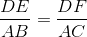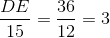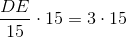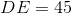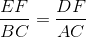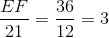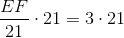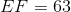The perimeter ofis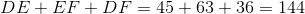.

### Example Question #3 : Acute / Obtuse Triangles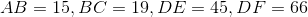What is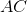?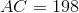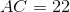It is impossible to tell from the information given.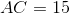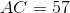Explanation:

By definition, since, all side lengths are in proportion.